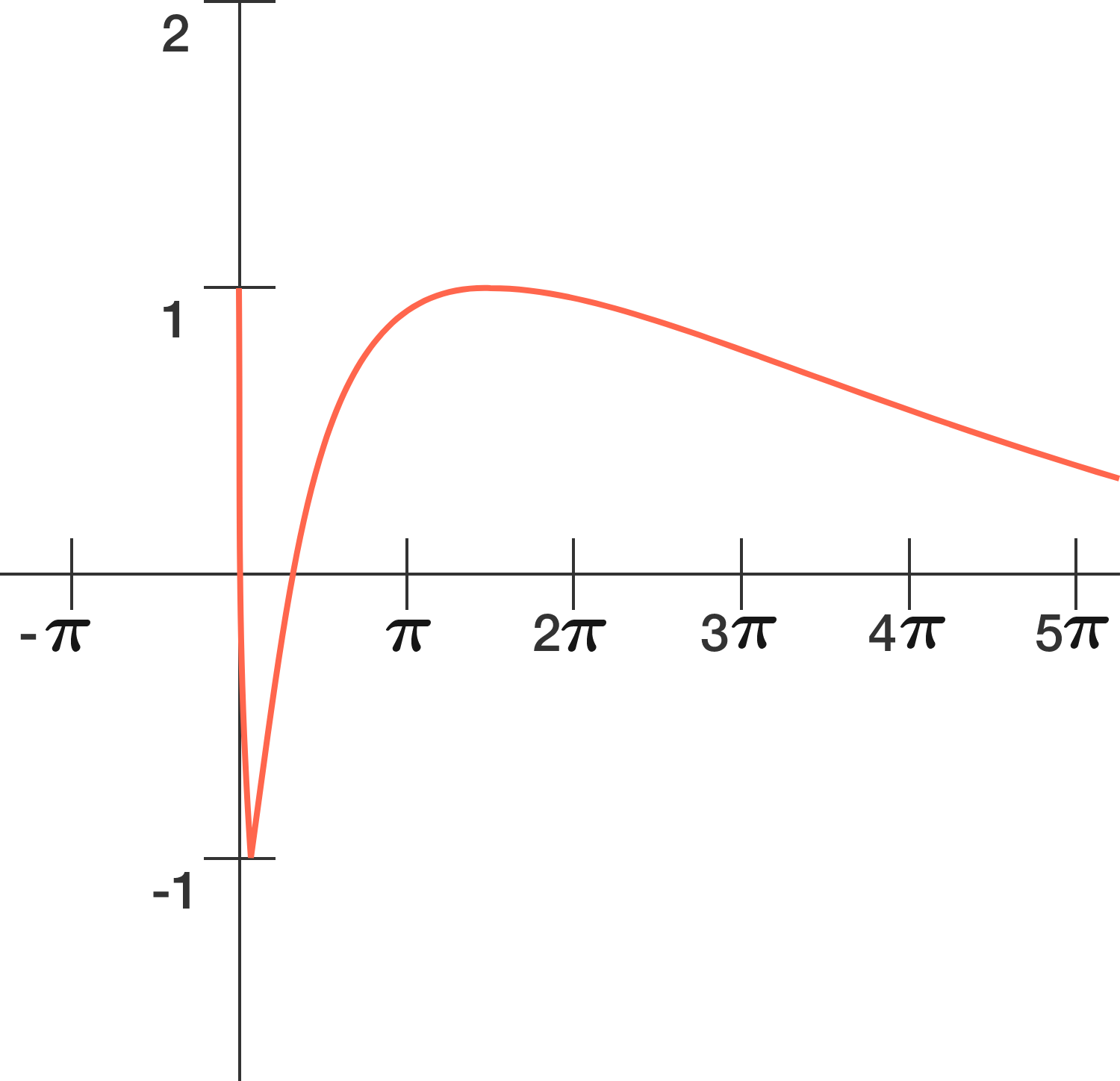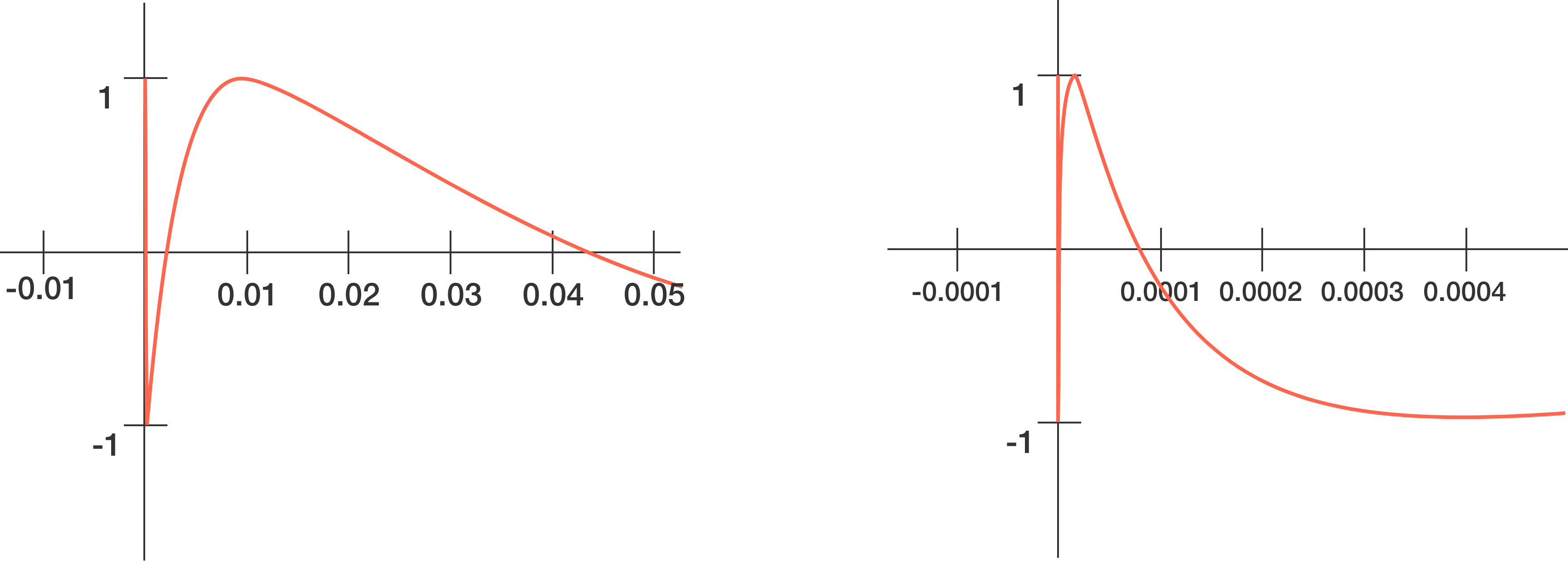# A very fickle function

Calculus Level 3The graph of $f(x) = \sin ( \ln x )$ (as shown above) looks innocent enough to noticeably oscillate as $x$ increases. However, as $x$ approaches $0$, the oscillations grow rapidly, making $f(x + \epsilon)$ vary greatly from $f(x)$ around this region, even at very infinitesimal values of $\epsilon$.That said, $f(x)$ will cross the $x$-axis for an infinite number of times from $x=0$ to $x=1$, creating several regions of the first quadrant enclosed by the curve and the $x$-axis.

If the sum of these regions is $A$, then determine $\big\lfloor 10^5 A \big\rfloor$.

×